Next: Theorems Up: k-Fibonacci numbers (this year) Previous: k-Fibonacci numbers (this year)   Contents

## Definitions

• The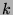-Fibonacci sequence (the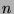th term of which is denoted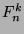) is defined recursively by the following statements:
• If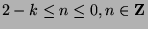, then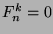.
•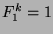.
•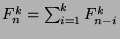,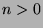.
•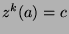means that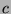has the following properties:
•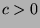.
• For all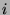such that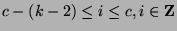, it follows that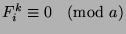.
•is the least positive integer satisfying these properties.
•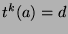means that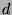has the following properties:
•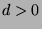• For allsuch that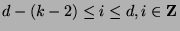, it follows that.
•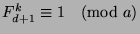.
•is the least positive integer satisfying these properties.
•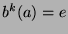means that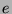has the following properties:
•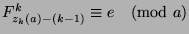.

Gregory Stoll 2000-04-08